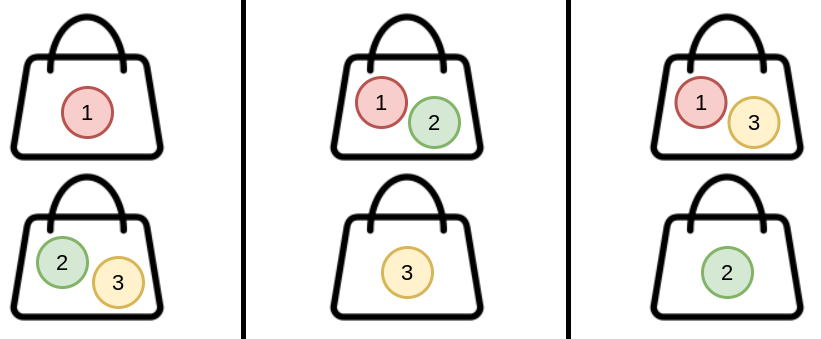Formatted question description: https://leetcode.ca/all/1692.html

# 1692. Count Ways to Distribute Candies

Hard

## Description

There are n unique candies (labeled 1 through n) and k bags. You are asked to distribute all the candies into the bags such that every bag has at least one candy.

There can be multiple ways to distribute the candies. Two ways are considered different if the candies in one bag in the first way are not all in the same bag in the second way. The order of the bags and the order of the candies within each bag do not matter.

For example, (1), (2,3) and (2), (1,3) are considered different because candies 2 and 3 in the bag (2,3) in the first way are not in the same bag in the second way (they are split between the bags (2) and (1,3)). However, (1), (2,3) and (3,2), (1) are considered the same because the candies in each bag are all in the same bags in both ways.

Given two integers, n and k, return the number of different ways to distribute the candies. As the answer may be too large, return it modulo 10^9 + 7.

Example 1:Input: n = 3, k = 2

Output: 3

Explanation: You can distribute 3 candies into 2 bags in 3 ways:

(1), (2,3)

(1,2), (3)

(1,3), (2)

Example 2:

Input: n = 4, k = 2

Output: 6

Explanation: You can distribute 4 candies into 2 bags in 7 ways:

(1), (2,3,4)

(1,2), (3,4)

(1,3), (2,4)

(1,4), (2,3)

(1,2,3), (4)

(1,2,4), (3)

(1,3,4), (2)

Example 3:

Input: n = 20, k = 5

Output: 206085257

Explanation: You can distribute 20 candies into 5 bags in 1881780996 ways. 1881780996 modulo 10^9 + 7 = 206085257.

Constraints:

• 1 <= k <= n <= 1000

## Solution

Use dynamic programming. Create a 2D array dp with k rows and n columns, where dp[i][j] represents the number of ways to distribute j + 1 candies into i + 1 bags.

Obviously, dp[i][j] = 0 when i > j. When there is only one bag, there is only one way to destribute the candies, so dp[j] = 1 for all 0 <= j < n.

For 1 <= i < k and i <= j < n, there is dp[i][j] = dp[i][j - 1] * (i - 1) + dp[i - 1][j - 1].

Finally, return dp[k - 1][n - 1].

class Solution {
public int waysToDistribute(int n, int k) {
final int MODULO = 1000000007;
long[][] dp = new long[k][n];
for (int j = 0; j < n; j++)
dp[j] = 1;
for (int i = 1; i < k; i++) {
for (int j = i; j < n; j++)
dp[i][j] = (dp[i][j - 1] * (i + 1) + dp[i - 1][j - 1]) % MODULO;
}
return (int) dp[k - 1][n - 1];
}
}